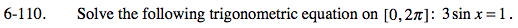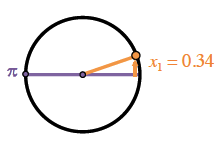### Home > PC > Chapter 6 > Lesson 6.3.1 > Problem6-110

6-110.

Solve the following trigonometric equation on [0, 2π]: 3sin x = 1. Homework Help ✎Solve for the sin(x).
Then use your calculator to find one of the angles.
Where and what is the other angle?